# Class 10 NCERT Solutions – Chapter 11 Constructions – Exercise 11.2

• Last Updated : 05 May, 2021

### Question 1. Draw a circle of radius 6 cm. From a point 10 cm away from its centre, construct the pair of tangents to the circle and measure their lengths?

Solution:

Construction Procedure:

The construction to draw a pair of tangents to the given circle is as follows.

Step 1. Draw a circle with radius = 6 cm with centre O.

Step 2. A point P can be constructed 10 cm away from centre O.

Step 3. The points O and P are then joined to form a line

Step 4. Draw the perpendicular bisector of the line OP.

Step 5. Construct M as the mid-point of the line segment PO.

Step 6. Using M as the centre, measure the length of the line segment MO

Step 7. Now using MO as the radius, draw a circle.

Step 8. The circle drawn with the radius of MO, intersect the previous circle at point Q and R.

Step 9. Join the line segments PQ and PR.

Step 10. Now, PQ and PR are the required tangents.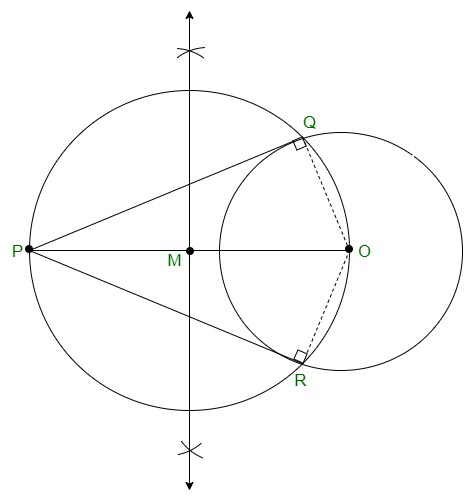Justification:

The construction of the given problem can be justified by proving that PQ and PR are the tangents to the circle of radius 6cm with centre O. This can be proved by joining OQ and OR which are represented in dotted lines.

From the construction, we can see that,

∠PQO is an angle in the semi-circle.

Every angle in a semi-circle is a right angle, therefore,

∴ ∠PQO = 90°

Now,

⇒ OQ ⊥ PQ

Since OQ is the radius of the circle with a radius of 6 cm, PQ must be a tangent of the circle. Similarly, we can also prove that PR is a tangent of the circle. Hence, justified.

### Question 2. Construct a tangent to a circle of radius 4 cm from a point on the concentric circle of radius 6 cm and measure its length. Also, verify the measurement by actual calculation?

Solution:

Construction Procedure:

For the specified circle, the tangent can be drawn as follows.

Step 1. Draw a circle of 4 cm radius with centre O.

Step 2. Taking O as the centre draw another circle of radius 6 cm.

Step 3. Locate a point P on this circle

Step 4. Join the points O and P to form a line segment OP.

Step 5. Construct the perpendicular bisector to the line OP, where M is the mid-point

Step 6. Taking M as its centre, draw a circle with MO as its radius

Step 7. The circle drawn with the radius OM, intersect the given circle at the points Q and R.

Step 8. Join the line segments PQ and PR.

Step 9. PQ and PR are the required tangents of the circle.

We can see that PQ and PR are of length 4.47 cm each.

Now, In ∆PQO,

Since PQ is a tangent,

∠PQO = 90°, PO = 6cm and QO = 4 cm

Applying Pythagoras theorem in ∆PQO, we obtain PQ2 + QO2 = PQ2

PQ2 + (4)2 = (6)2

=> PQ2 + 16 = 36

=> PQ2 = 36 − 16

=> PQ2 = 20

PQ = 2√5

PQ = 4.47 cm

Therefore, the tangent length PQ = 4.47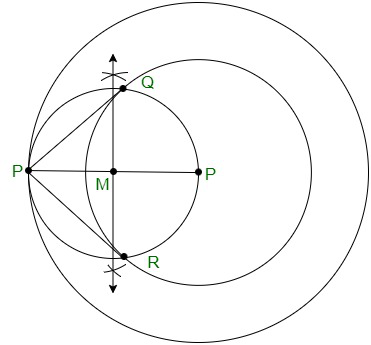Justification:

It can be proved that PQ and PR are the tangents to the circle of radius 4 cm with centre O.

Proof,

Join OQ and OR represented in dotted lines. Now,

∠PQO is an angle in the semi-circle.

Every angle in a semi-circle is a right angle, therefore, ∠PQO = 90° s.t

⇒ OQ ⊥ PQ

Since OQ is the radius of the circle with a radius of 4 cm, PQ must be a tangent of the circle. Similarly, we can prove that PR is a tangent of the circle.

### Question 3. Draw a circle of radius 3 cm. Take two points P and Q on one of its extended diameters each at a distance of 7 cm from its centre. Draw tangents to the circle from these two points P and Q?

Solution:

Construction Procedure:

The tangent for the given circle can be constructed as follows.

Step 1. Construct a circle with a radius of 3cm with centre O.

Step 2. Draw a diameter of a circle that extends 7 cm from the centre O of the circle and mark the endpoints as P and Q.

Step 3. Draw the perpendicular bisector of the constructed line segment PO.

Step 4. Mark the midpoint of PO as M.

Step 5. Draw another circle with M as centre and MO as its radius

Step 6. Now join the points PA and PB in which the circle with radius MO intersects the circle of circle 3cm.

Step 7. PA and PB are the required tangents of the circle.

Step 8. From that, QC and QD are the required tangents from point Q.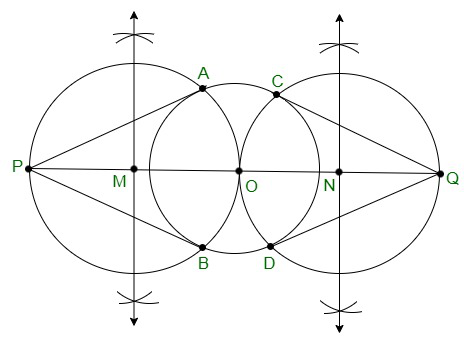Justification:

The construction of the given problem can be justified by proving that PQ and PR are the tangents to the circle of radius 3 cm with centre O.

Proof,

Join OA and OB. Now,

∠PAO is an angle in the semi-circle, which is equal to 90 degrees.

∴ ∠PAO = 90° s.t

⇒ OA ⊥ PA

Since OA is the radius of the circle with a radius of 3 cm, PA must be a tangent of the circle.

PB, QC, and QD are the tangent of the circle [by similar proof]. Hence, justified.

### Question 4. Draw a pair of tangents to a circle of radius 5 cm which is inclined to each other at an angle of 60°?

Solution:

Construction Procedure:

The tangents can be constructed in the following manner:

Step 1. Draw a circle with a centre O of the radius of 5 cm.

Step 2. Construct any arbitrary point Q on the circumference of the circle and join the line segment OQ.

Step 3. Also, draw a perpendicular to QP at point Q.

Step 4. Draw a radius OR, making an angle of 120° i.e(180°−60°) with OQ.

Step 5. Draw a perpendicular to the line RP at point R.

Step 6. Both the perpendicular bisectors intersect at P.

Step 7. Therefore, PQ and PR are the required tangents at an angle of 60°.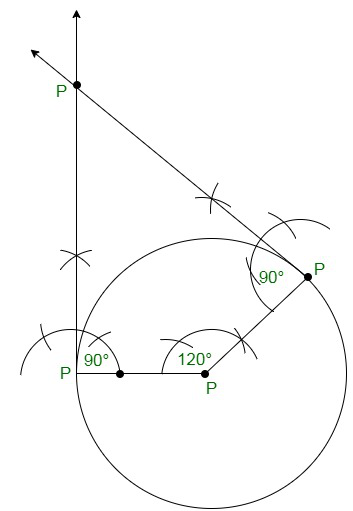Justification:

The construction can be justified by proving that ∠QPR = 60°

We have,

∠OQP = 90°, ∠ORP = 90°

Also ∠QOR = 120°

We know that, the summation of the interior angles of a quadrilateral = 360°

Substituting values,

∠OQP + ∠QOR + ∠ORP + ∠QPR = 360o

=> 90° + 120° + 90° + ∠QPR = 360°

Calculating, we get, ∠QPR = 60°

Hence, justified.

### Question 5. Draw a line segment AB of length 8 cm. Taking A as the centre, draw a circle of radius 4 cm, and taking B as centre, draw another circle of radius 3 cm. Construct tangents to each circle from the centre of the other circle?

Solution:

Construction Procedure:

The tangent for the given circle can be constructed as follows.

Step 1. Construct a line segment named AB = 8 cm.

Step 2. Taking A as the centre and draw a circle of a radius of 4 cm.

Step 3. Taking B as centre, draw another circle of radius 3 cm.

Step 4. Draw the perpendicular bisector of the line AB with M as the midpoint.

Step 5. Taking M as the centre, draw another circle with the radius of MA or MB which intersects the circle at the points P, Q, R, and S.

Step 6. Join the line segments AR, AS, BP, and BQ respectively.

Step 7. The required tangents are AR, AS, BP, and BQ.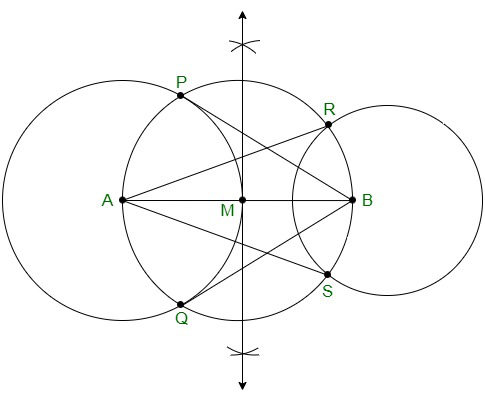Justification:

The construction can be justified by proving that AS and AR are the tangents of the circle with centre B and BP and BQ are the tangents of the circle with a circle centered at A.

Proof,

Join AP, AQ, BS, and BR.

∠ASB is an angle in the semi-circle.

∴ ∠ASB = 90°

⇒ BS ⊥ AS

Now, BS is the radius of the circle. Therefore, AS must be a tangent of the circle. Similarly, AR, BP, and BQ are the required tangents of the given circle.

### Question 6. Let ABC be a right triangle in which AB = 6 cm, BC = 8 cm and ∠ B = 90°. BD is perpendicular from B on AC. The circle through B, C, D is drawn. Construct the tangents from A to this circle?

Solution:

Construction Procedure:

The tangent for the given circle can be constructed as follows

Step 1. Draw a line segment with base BC = 8cm

Step 2. Construct an angle of 90° at point B, s.t ∠ B = 90°.

Step 3. Taking B as centre, draw an arc of 6cm.

Step 4. Mark the point of intersection as A.

Step 5. Join the line segment AC.

Step 6. Now, we have ABC as the required triangle.

Step 7. Now, construct the perpendicular bisector to the line BC with the midpoint as E.

Step 8. Taking E as the centre, draw a circle with BE or EC as the radius.

Step 9. Join A and E to form a line segment.

Step 10. Now, again draw the perpendicular bisector to the line AE and the midpoint is taken as M

Step 11. Taking M as the centre, draw a circle with AM or ME as the radius.

Step 12. Both the circles intersect at points B and Q.

Step 13. Join points A and Q to form a line segment.

Step 14. Therefore, AB and AQ are the required tangents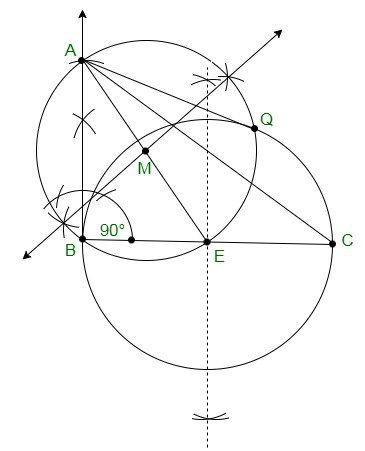Justification:

The construction can be justified by proving that AG and AB are the tangents to the circle.

Proof,

Join EQ.

∠AQE is an angle in the semi-circle.

∴ ∠AQE = 90°

Now, ⇒ EQ⊥ AQ

Since EQ is the radius of the circle, AQ will be a tangent of the circle. Also, ∠B = 90°

⇒ AB ⊥ BE

Since BE is the radius of the circle, AB has to be a tangent of the circle. Hence, justified.

### Question 7. Draw a circle with the help of a bangle. Take a point outside the circle. Construct the pair of tangents from this point to the circle?

Solution:

Construction Procedure:

The required tangents can be constructed on the given circle as follows.

Step 1. Draw an arbitrary circle. Mark its centre as O.

Step 2. Draw two non-parallel chords such as AB and CD.

Step 3. Draw the perpendicular bisector of AB and CD

Step 4. Taking O as the centre where both the perpendicular bisectors AB and CD intersect.

Step 5. Take a point P outside the circle.

Step 6. Join the points O and P to form a line segment.

Step 7. Now draw the perpendicular bisector of the line PO and mark its midpoint as M.

Step 8. Taking M as centre and MO as the radius draw a circle.

Step 9.  Both the circles intersect at the points Q and R.

Step 10. Now join PQ and PR.

Step 11. Therefore, PQ and PR are the required tangents.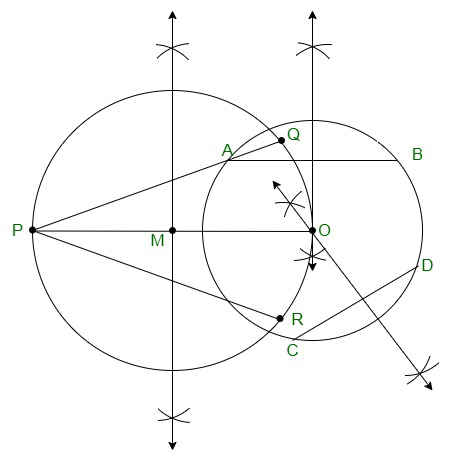Justification:

The construction can be justified by proving that PQ and PR are the tangents to the circle. The perpendicular bisector of any chord of the circle passes through the centre. Now, join the points OQ and OR.

Both the perpendicular bisectors intersect at the centre of the circle. Since ∠PQO is an angle in the semi-circle.

∴ ∠PQO = 90°

⇒ OQ⊥ PQ

Since OQ is the radius of the circle, PQ has to be a tangent of the circle. Similarly,

∴ ∠PRO = 90°

⇒ OR ⊥ PO

Similarly, since OR is the radius of the circle, PR has to be a tangent of the circle. Therefore, PQ and PR are the tangents of a circle.

My Personal Notes arrow_drop_up
Recommended Articles
Page :